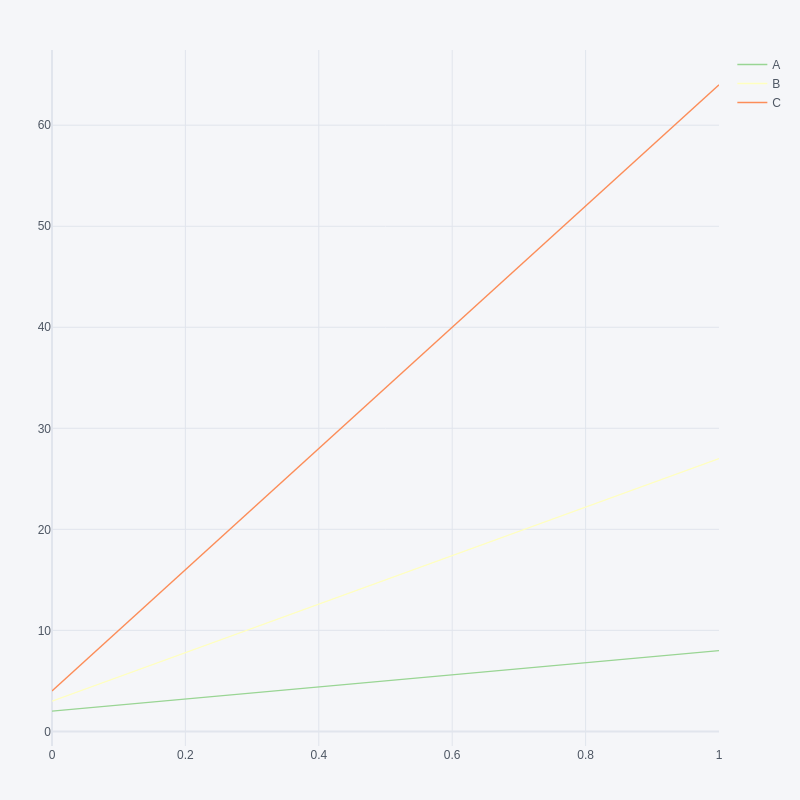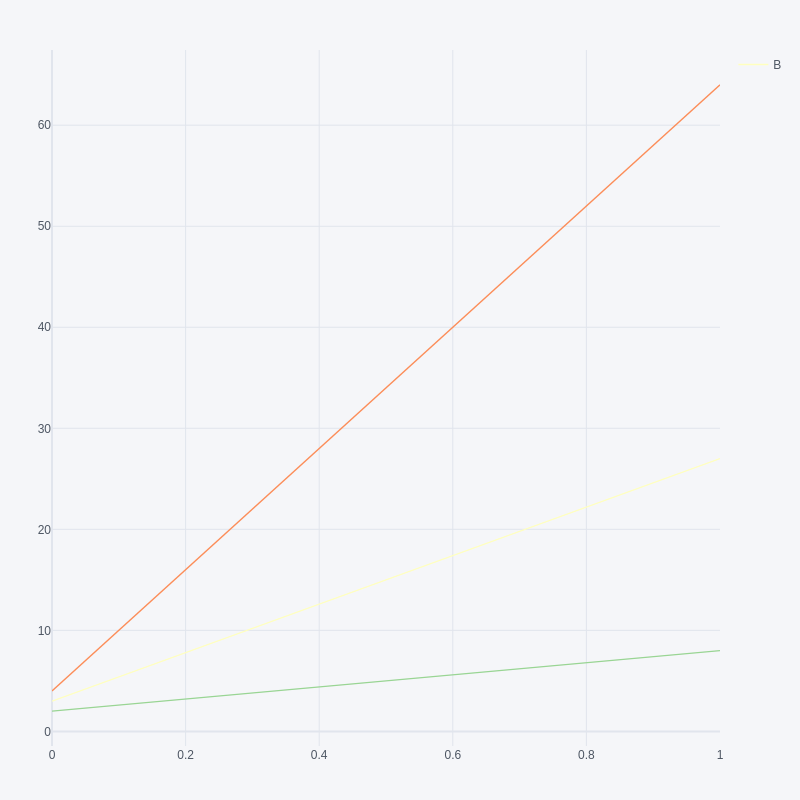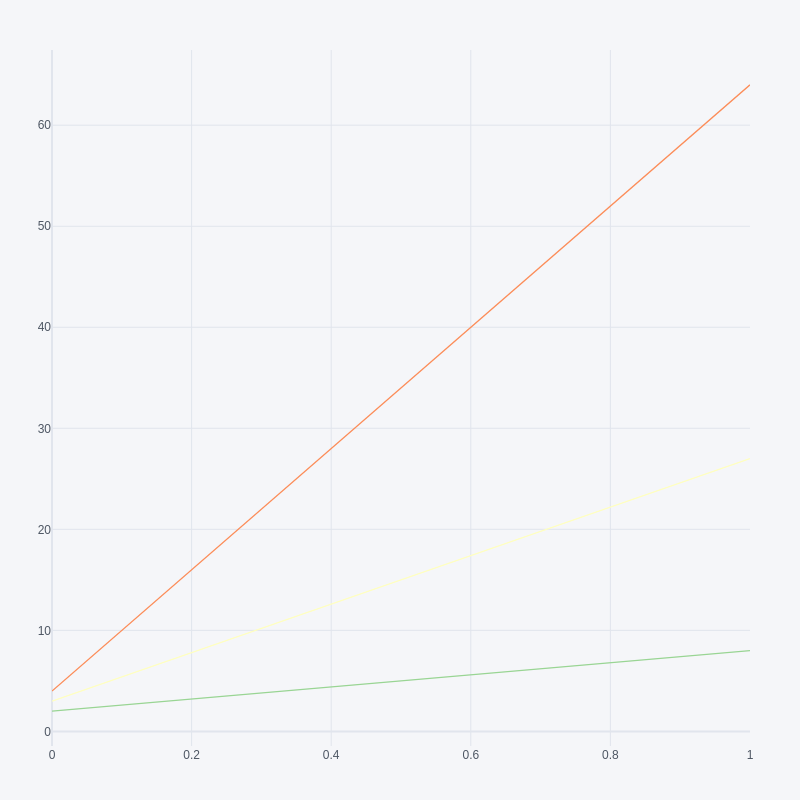GeeksforGeeks App
Open AppBrowser
Continue

# Hide legend entries in a plotly figure in Python

In this article, we are going to see how to hide legend entries in a plotly figure using Python.

The Fig below shows the plot without hiding the legend entries:## Method 1: Setting showlegend property by the name of the trace

Here we are going to set showlegend property to remove the legend entries in a plotly figure.

## Python3

 `import` `pandas as pd``import` `plotly.offline as py``import` `plotly.graph_objs as go``import` `cufflinks as cf``cf.go_offline()`` ` `df ``=` `pd.DataFrame(data``=``[[``2``, ``3``, ``4``], [``8``, ``27``, ``64``]],``                  ``columns``=``[``'A'``, ``'B'``, ``'C'``])`` ` `# get figure property``fig ``=` `df.iplot(kind``=``'scatter'``, asFigure``=``True``)`` ` `# set showlegend property by name of trace``for` `trace ``in` `fig[``'data'``]:``    ``if``(trace[``'name'``] !``=` `'B'``):``        ``trace[``'showlegend'``] ``=` `False`` ` `# generate webpage``py.plot(fig)`

Output:## Method 2: Using the update function, remove all the entries

Here we are going to use the update function to remove all the entries

## Python3

 `import` `pandas as pd``import` `plotly.offline as py``import` `plotly.graph_objs as go``import` `cufflinks as cf``cf.go_offline()`` ` `df ``=` `pd.DataFrame(data``=``[[``2``, ``3``, ``4``], [``8``, ``27``, ``64``]], ``                  ``columns``=``[``'A'``, ``'B'``, ``'C'``])`` ` `# get figure property``fig ``=` `df.iplot(kind``=``'scatter'``, asFigure``=``True``)`` ` `# set layout_showlegend=False``fig.update(layout_showlegend``=``False``)`` ` `# generate webpage``py.plot(fig)`

Output:My Personal Notes arrow_drop_up